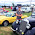## Monday, 25 January 2016

### Proving two wrongs does not make a right

First way

Wrong is a negative number
Right is a positive number

1 wrong = -1
1 wrong = -1

1 wrong + 1 wrong = 2 wrongs
-1 + -1 = -2 = negative = 2 wrongs

1 right + 1 right = 2 rights
1 + 1 = 2 = positive = 2 rights

Second way

Wrong is false
Right is true

In binary false = 0
In binary true  = 1

wrong + wrong = 2 wrongs
0 AND 0 = 0 = wrong
1 AND 1 = 1 = right

Have any more ways?

Comment if you know any other ways of doing this!

1.Wrong is negative, Right is positive. 2 Wrongs = -1*-1 = 1, which is positive.

So 2 Wrongs make a Right !!

1.Yeah, That was my first way, but I decided to post using addition so that people wouldn't do 2 wrongs ;)

2.This comment has been removed by the author.